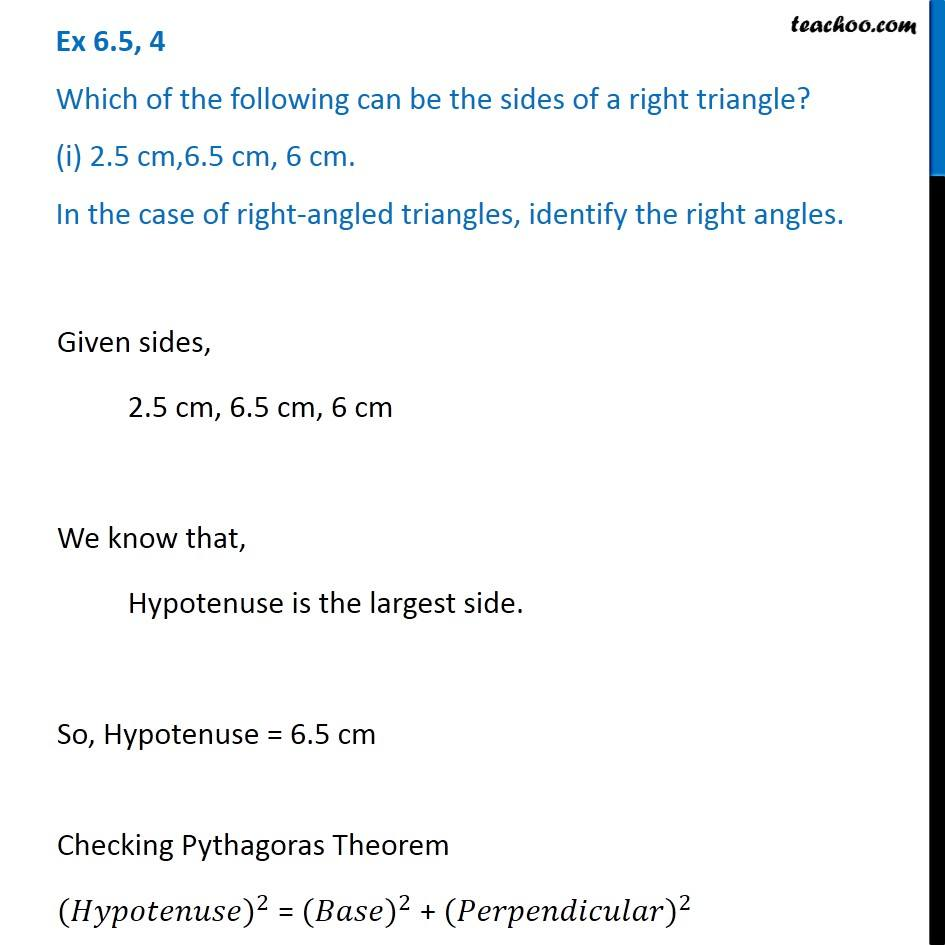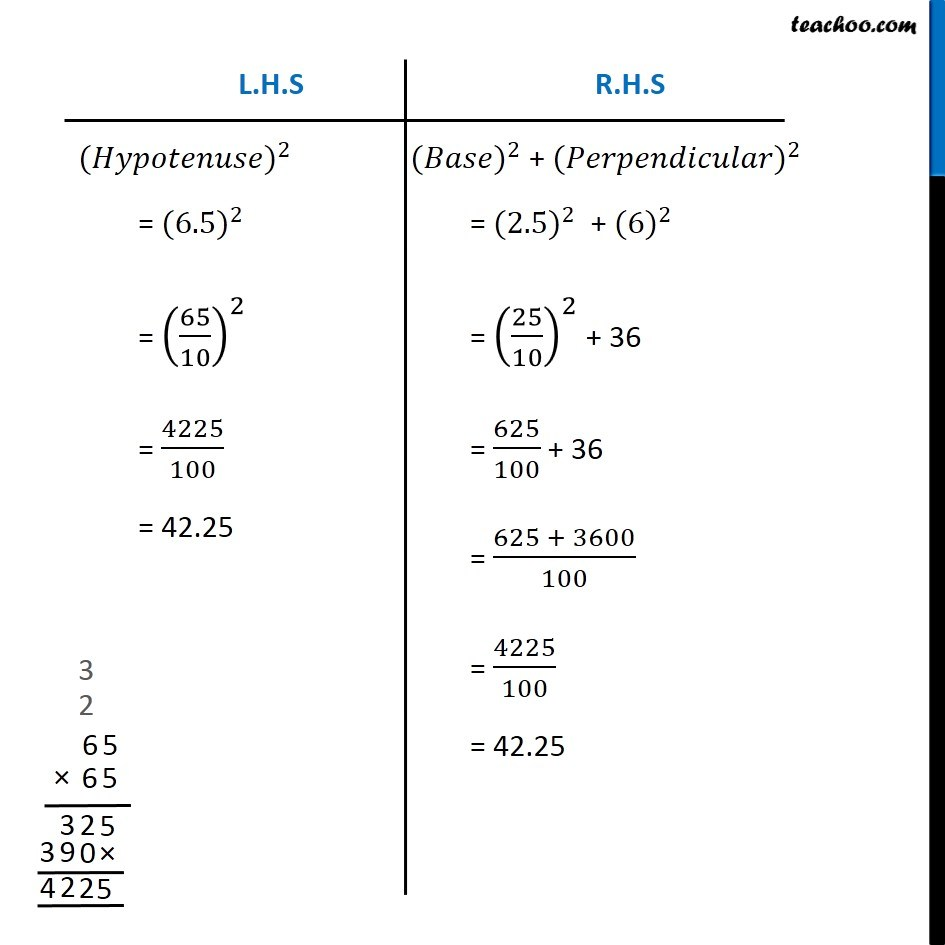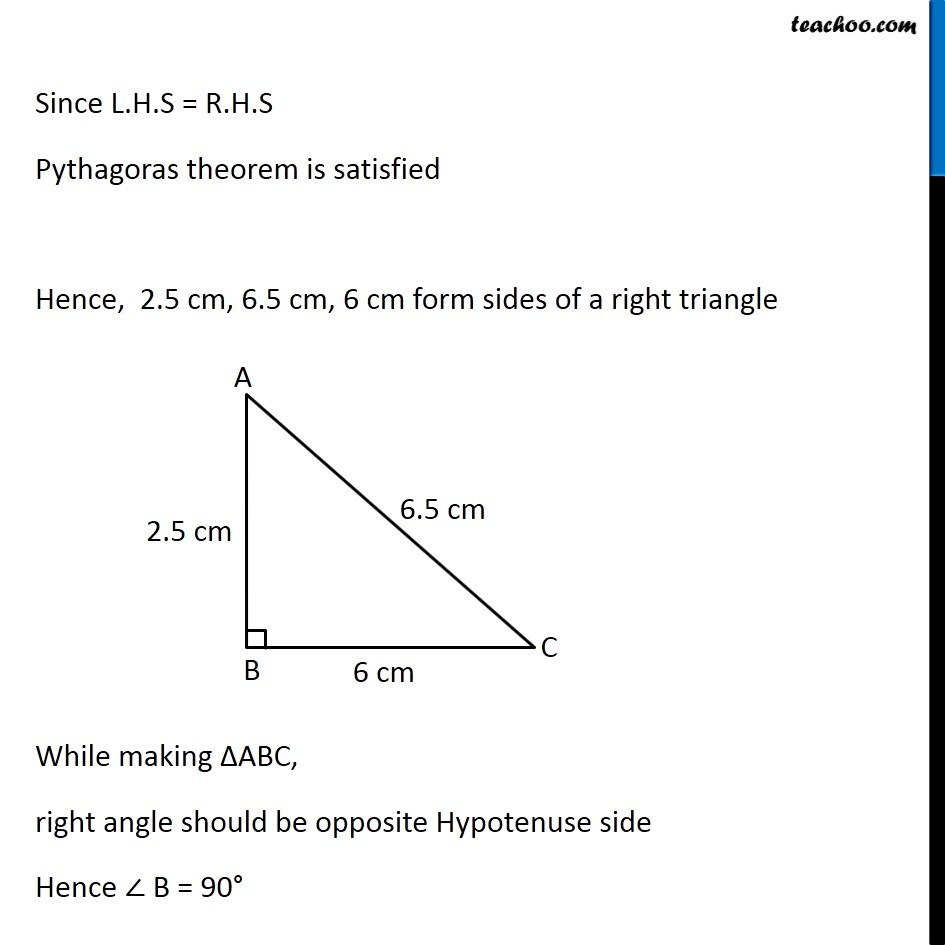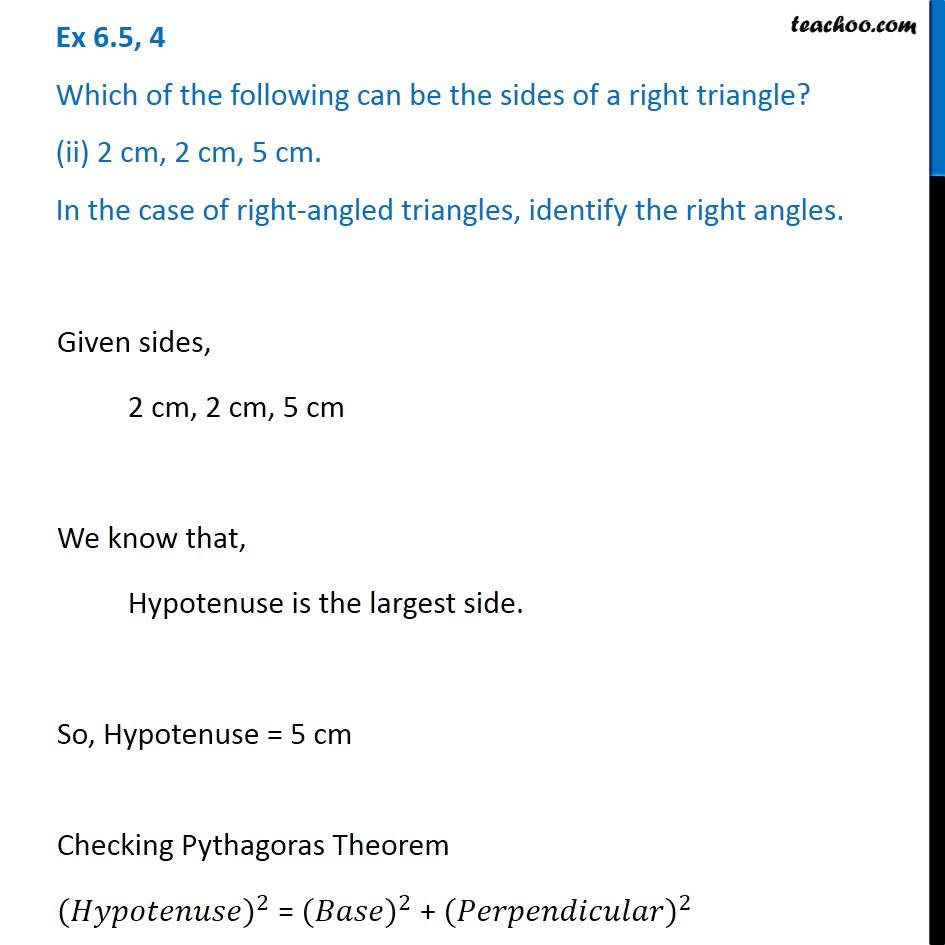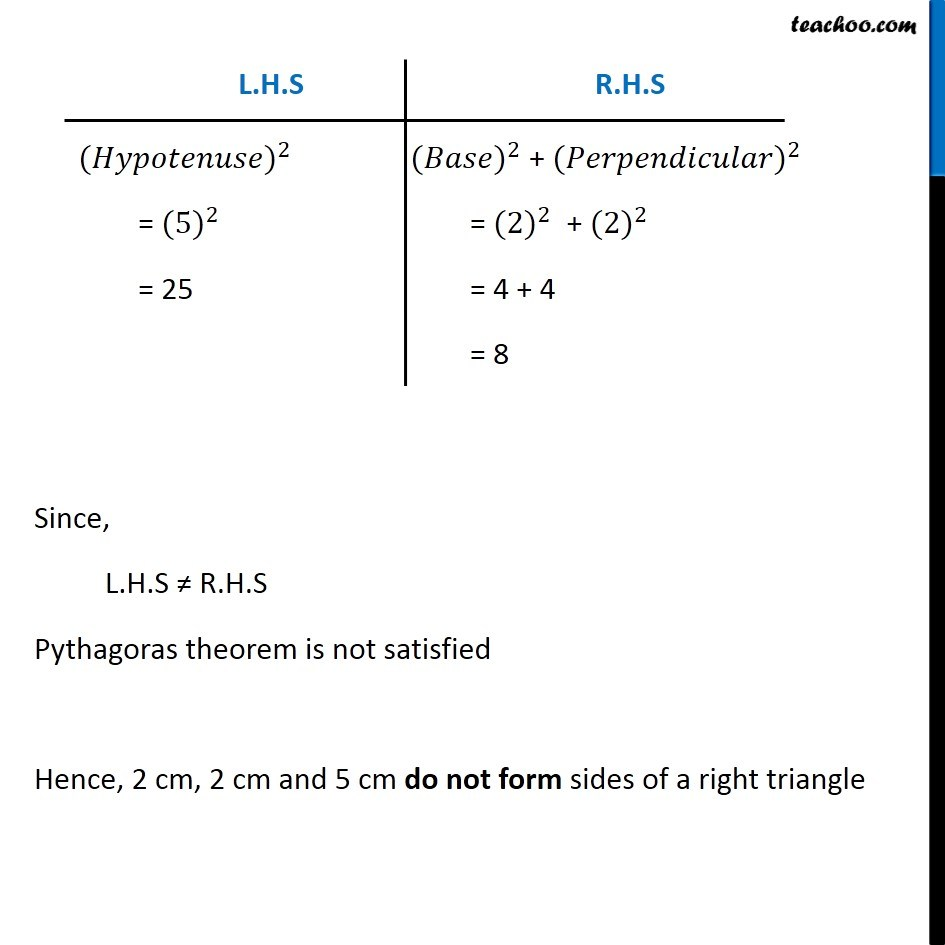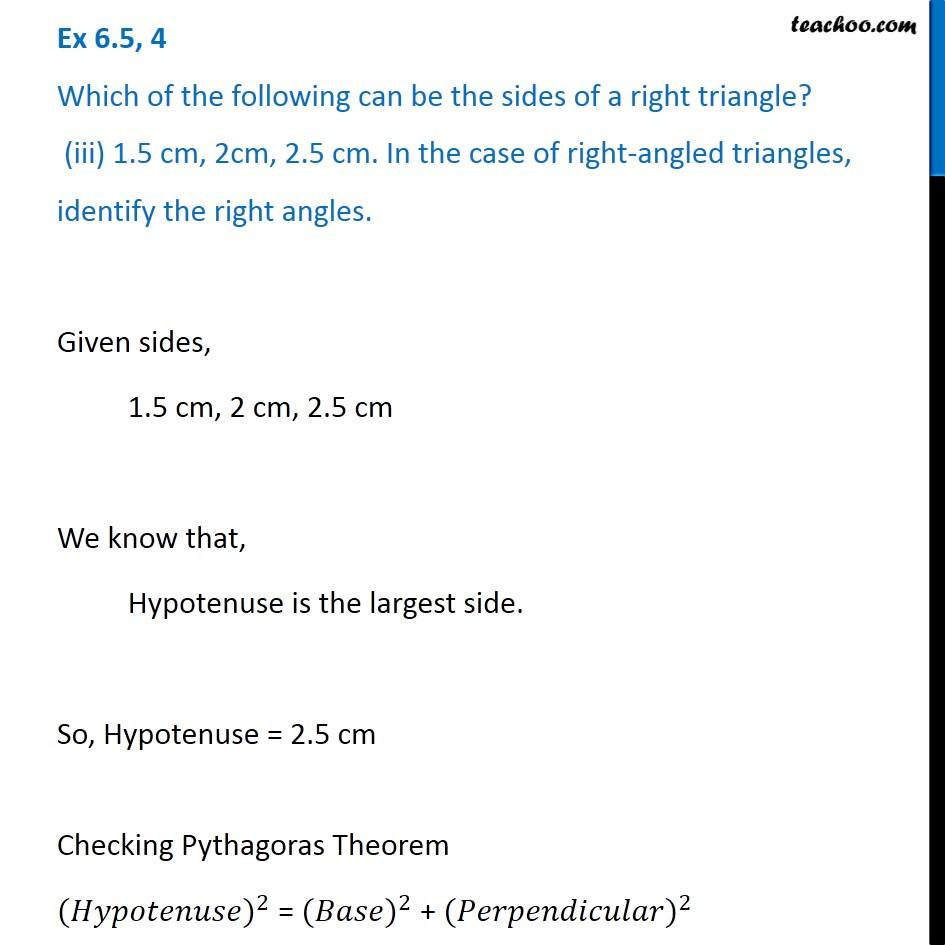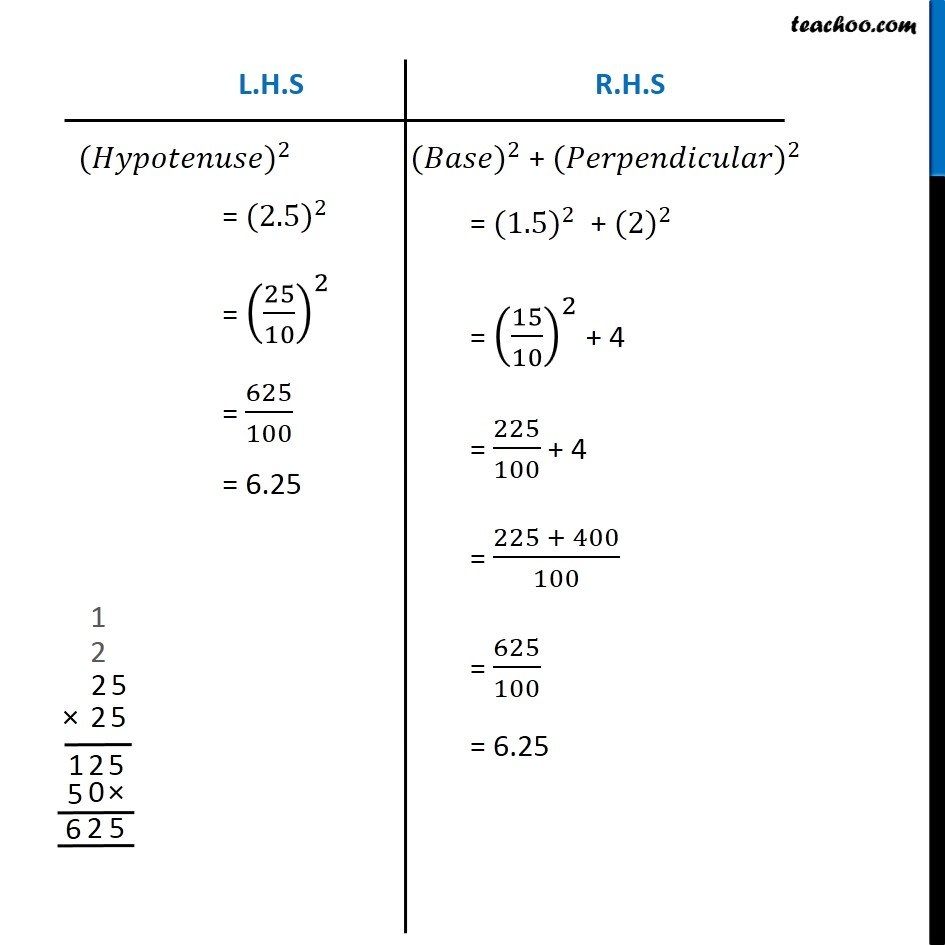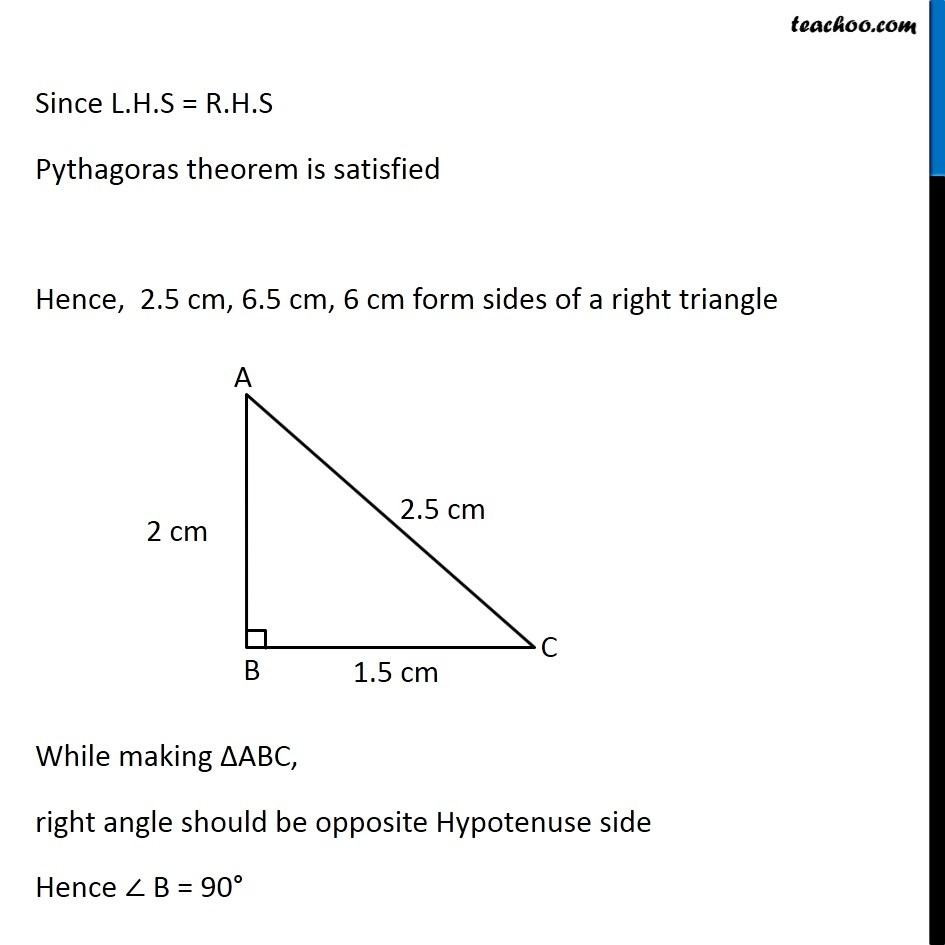Subscribe to our Youtube Channel - https://you.tube/teachoo

1. Chapter 6 Class 7 Triangle and its Properties
2. Serial order wise
3. Ex 6.5

Transcript

Ex 6.5, 4 Which of the following can be the sides of a right triangle? (i) 2.5 cm,6.5 cm, 6 cm. In the case of right-angled triangles, identify the right angles. Given sides, 2.5 cm, 6.5 cm, 6 cm We know that, Hypotenuse is the largest side. So, Hypotenuse = 6.5 cm Checking Pythagoras Theorem 〖(𝐻𝑦𝑝𝑜𝑡𝑒𝑛𝑢𝑠𝑒)〗^2 = 〖(𝐵𝑎𝑠𝑒)〗^2 + 〖(𝑃𝑒𝑟𝑝𝑒𝑛𝑑𝑖𝑐𝑢𝑙𝑎𝑟)〗^2 〖(𝐻𝑦𝑝𝑜𝑡𝑒𝑛𝑢𝑠𝑒)〗^2 = 〖(6.5)〗^2 = (65/10)^2 = 4225/100 = 42.25 〖(𝐵𝑎𝑠𝑒)〗^2 + 〖(𝑃𝑒𝑟𝑝𝑒𝑛𝑑𝑖𝑐𝑢𝑙𝑎𝑟)〗^2 = 〖(2.5)〗^2 + 〖(6)〗^2 = (25/10)^2 + 36 = 625/100 + 36 = (625 + 3600)/100 = 4225/100 = 42.25 Since L.H.S = R.H.S Pythagoras theorem is satisfied Hence, 2.5 cm, 6.5 cm, 6 cm form sides of a right triangle While making ∆ABC, right angle should be opposite Hypotenuse side Hence ∠ B = 90° Ex 6.5, 4 Which of the following can be the sides of a right triangle? (ii) 2 cm, 2 cm, 5 cm. In the case of right-angled triangles, identify the right angles. Given sides, 2 cm, 2 cm, 5 cm We know that, Hypotenuse is the largest side. So, Hypotenuse = 5 cm Checking Pythagoras Theorem 〖(𝐻𝑦𝑝𝑜𝑡𝑒𝑛𝑢𝑠𝑒)〗^2 = 〖(𝐵𝑎𝑠𝑒)〗^2 + 〖(𝑃𝑒𝑟𝑝𝑒𝑛𝑑𝑖𝑐𝑢𝑙𝑎𝑟)〗^2 〖(𝐻𝑦𝑝𝑜𝑡𝑒𝑛𝑢𝑠𝑒)〗^2 = 〖(5)〗^2 = 25 〖(𝐵𝑎𝑠𝑒)〗^2 + 〖(𝑃𝑒𝑟𝑝𝑒𝑛𝑑𝑖𝑐𝑢𝑙𝑎𝑟)〗^2 = 〖(2)〗^2 + 〖(2)〗^2 = 4 + 4 = 8 Since, L.H.S ≠ R.H.S Pythagoras theorem is not satisfied Hence, 2 cm, 2 cm and 5 cm do not form sides of a right triangle Ex 6.5, 4 Which of the following can be the sides of a right triangle? (iii) 1.5 cm, 2cm, 2.5 cm. In the case of right-angled triangles, identify the right angles. Given sides, 1.5 cm, 2 cm, 2.5 cm We know that, Hypotenuse is the largest side. So, Hypotenuse = 2.5 cm Checking Pythagoras Theorem 〖(𝐻𝑦𝑝𝑜𝑡𝑒𝑛𝑢𝑠𝑒)〗^2 = 〖(𝐵𝑎𝑠𝑒)〗^2 + 〖(𝑃𝑒𝑟𝑝𝑒𝑛𝑑𝑖𝑐𝑢𝑙𝑎𝑟)〗^2 〖(𝐵𝑎𝑠𝑒)〗^2 + 〖(𝑃𝑒𝑟𝑝𝑒𝑛𝑑𝑖𝑐𝑢𝑙𝑎𝑟)〗^2 = 〖(1.5)〗^2 + 〖(2)〗^2 = (15/10)^2 + 4 = 225/100 + 4 = (225 + 400)/100 = 625/100 = 6.25 Since L.H.S = R.H.S Pythagoras theorem is satisfied Hence, 2.5 cm, 6.5 cm, 6 cm form sides of a right triangle While making ∆ABC, right angle should be opposite Hypotenuse side Hence ∠ B = 90°

Ex 6.5